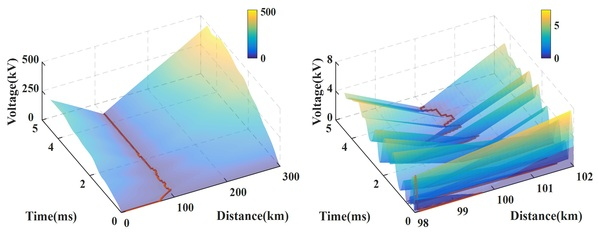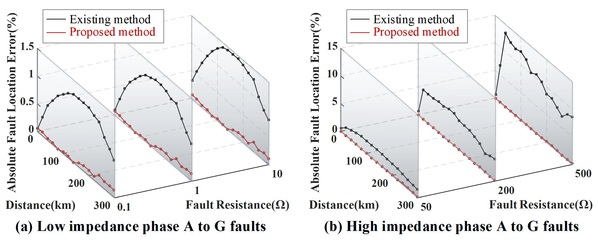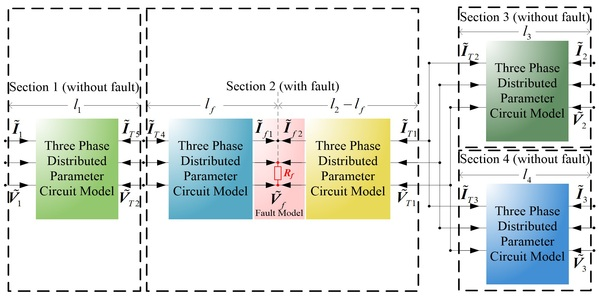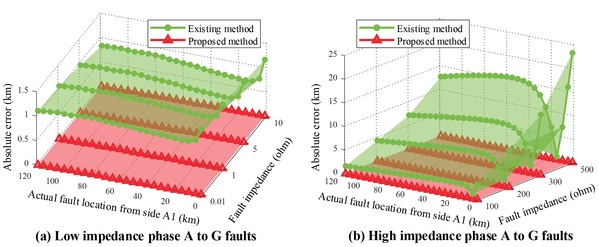# SIST Liu Yu’s Research Group Publishes Two Papers in IEEE Transactions on Power Delivery

ON2020-03-02TAG: ShanghaiTech UniversityCATEGORY: Published Research

Recently, Professor Liu Yu’s group from Center for Intelligent Power and Energy Systems (CiPES) of SIST, proposed two accurate fault location methods on long and complex transmission lines. These two papers were published online in IEEE Transactions on Power Delivery, the best peer-reviewed journal in the field of power system protection, transmission and distribution.

High-voltage long-distance power transmission is able to transmit huge amount of clean energy with low loss, and therefore enable increasing utilization of the clean energy. However, faults may sometimes occur on transmission lines. After isolation of the faulted line, accurate fault location is very essential since it can minimize the time spent searching for the fault, reduce power outage time and improve the reliability of power supply. With the development of modern power systems, transmission lines are evolving with increased complexity such as long distance, non-homogeneous, asymmetry, multi-terminal and mutual coupling, which make it more challenging to accurately determine the location of the fault.

The paper entitled “Time-domain Transmission Line Fault Location Method with Full Consideration of Distributed Parameters and Line Asymmetry” achieves accurate fault location by accurately calculating the voltage distribution along the transmission line during the fault in time domain. The paper proposes a new numerical scheme specifically for the fault location problem, to solve the partial differential equations (PDEs) corresponding to the complex and asymmetrical multi-phase transmission line. The PDEs describe the physical laws that the line voltages and currents should obey, with full consideration of distributed parameters and line asymmetry. Based on strict mathematical derivation, the stability and the minimum error of the proposed numerical scheme can be ensured with the suggested selection of the numerical intervals. Numerical experiments verify that the proposed numerical scheme and the fault location method achieve more accurate fault location results with extremely short data window (5 ms) and a relatively low sampling rate (4 kHz) compared to the existing time domain fault location methods. First year Ph.D. student Lu Dayou is the first author, Prof. Liu Yu is the corresponding author, Prof.  Liao Qifeng is the co-author, ShanghaiTech University is the first completion unit, and Shanghai Jiaotong University is the cooperative unit. The work is supported in part by the National Natural Science Foundation of China and Shanghai Pujiang Program.Figure. 1. Estimated voltage distribution along the transmission line during faults

(time window: 5ms, fault location:100 km)Figure. 2. Fault location results comparison: the existing time domain method and the proposed method

The paper entitled “Fault Location Algorithm for Non-Homogeneous Transmission Lines Considering Line Asymmetry” achieves accurate fault location by solving the state equation of the transmission line in phasor domain. Compared to the transmission line model in the time domain, the line model in phasor domain can be represented through ordinary differential equations (instead of partial differential equations) with the assumption of sinusoidal excitation and steady state. Therefore, the fault location problem is much simplified. In this paper, the generalized compact model of each homogeneous line section is derived by analytically solving the ordinary differential equations. A systematic methodology is proposed to formulate the model of the overall non-homogeneous transmission line by combining the generalized compact model of each section. Afterwards, the location of the fault is identified using the state estimation algorithm. Numerical experiments demonstrate that the proposed method presents higher accuracy compared to existing methods and has no further assumptions on the topology of the transmission line. Prof. Liu Yu is the first author, second year graduate student Wang Binglin is the second author, Prof. Fu Minfan is the co-author, ShanghaiTech University is the first completion unit, and Shanghai Jiaotong University is the cooperative unit. The work is supported in part by the National Natural Science Foundation of China and Shanghai Pujiang Program.Figure 3. Model of an example three-terminal non-homogeneous line with faultFigure 4. Fault location results comparison: the existing phasor domain method and the proposed method